# Shear Area Derivation

## Shear Area Derivation

It is here outlined how BHoM calculates shear area for a section
Shear Area formula used for calculation: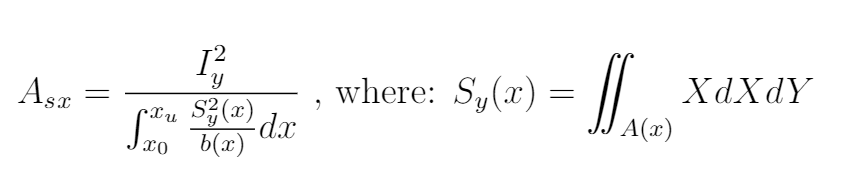And A(x) is defined as all the points less than x within the region A.

Moment of inertia is know and hence the denominator will be the focus.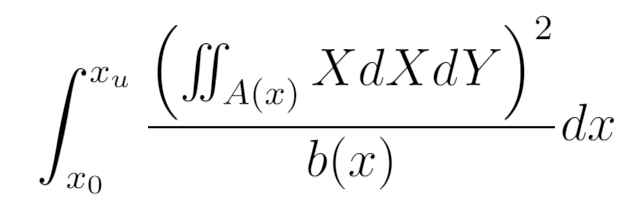Sy for an area can be calculated for a region by its bounding curves with Greens Therom: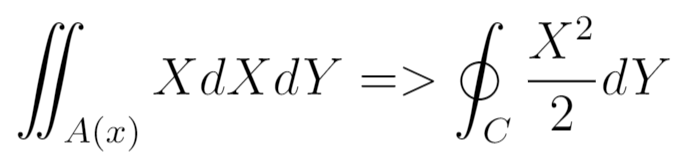which for line segments is: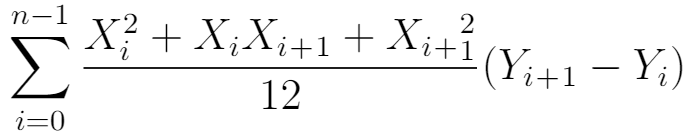And while calculating this for the entire region as line segments is easy, we want to have the regions size as a variable of x.
So we make some assumptions about the region we are evaluating. * Its upper edge is always on the X-axis * No overhangs

i.e. its thickness at any x is defined by its lower edge,
achieved by using WetBlanketIntegration()
Example: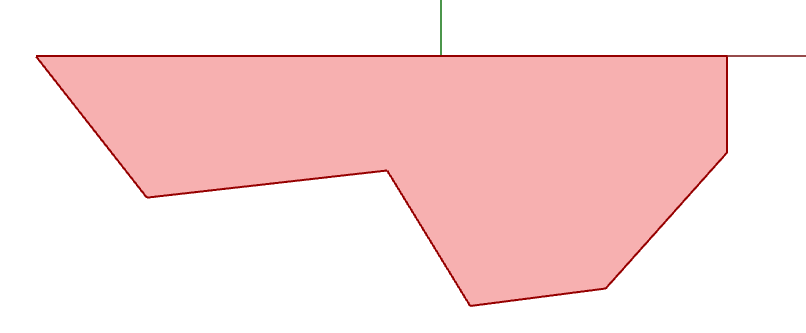We will then calculate the solution for each line segment from left to right.
This is important as Sy is dependent on everything to the left of it.

We then split the solution for Sy into three parts: * S0, The partial solution for every previous line, i.e. sum until current * The current line segment with variable t * A closing line segment with variable t, connects the end of the current line segment to the X-axis

Closing along the X-axis is not needed as the horizontal solution is always zero.
Visual representation of the area it works on: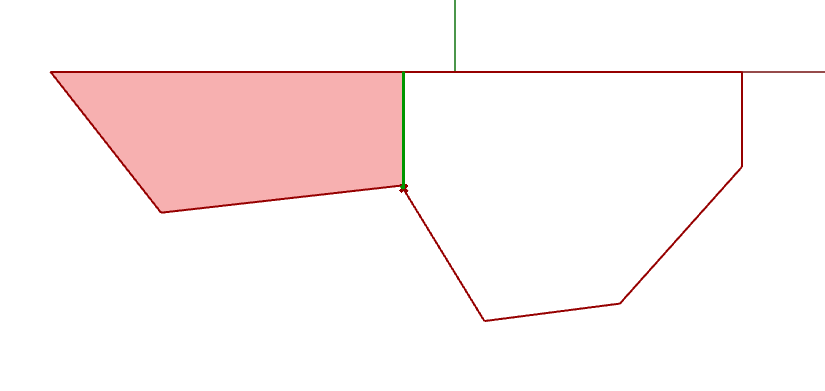We will now want to define all variables in relation to t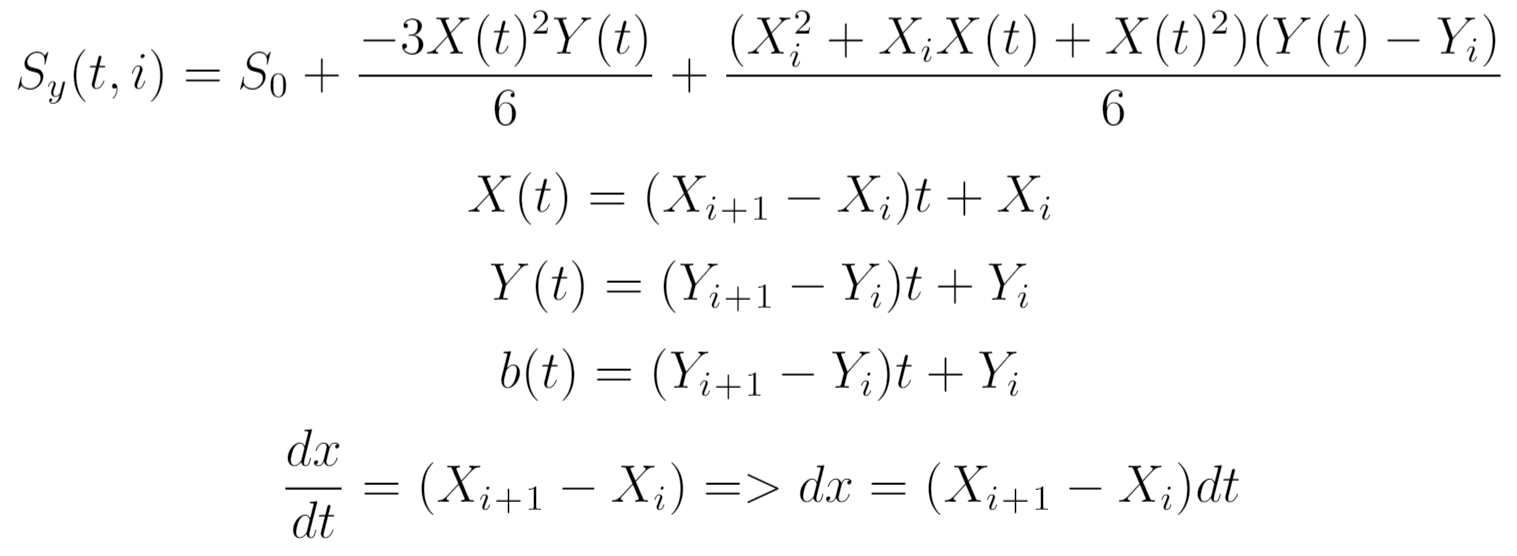And then plug everything into the integral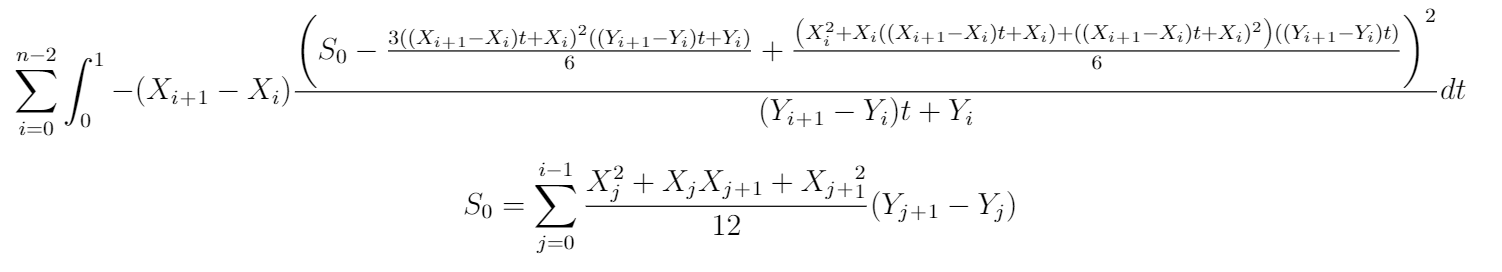### To summarise the practical proccess

1. The region will be converted to the right format by WetBlanketInterpetation()
2. The integral is evaluated for the first line segment and added to the results sum
3. The S0 value for the first line segment is calculated and added to the S_0 sum
4. step 2 and 3 repeats for every line except the last
5. The squared Moment of inertia is then divided by the result

Last update: September 20, 2023